# How to Highlight Cell or Row If Date Is In Current Day/Week/Month in Excel

This post will guide you how to highlight cell if date is the current day or is in the current week or month in Excel. How do I highlight row if date is in current week or month with conditional formatting in Excel 2013/2016.

## Highlight Cell if Date is in Current Day/Week/Month

To highlight the cell of current day or the date is in the current week or month, you can do the following steps:

#1 select the range of cells that you want to highlight date.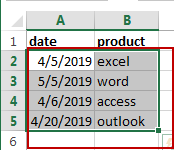#2 go to HOME tab, click Conditional Formatting command under Styles group, and click Highlight Cells Rules menu from the drop down menu list, then select A Date Occurring sub menu. And the A Date Occurring dialog will appear.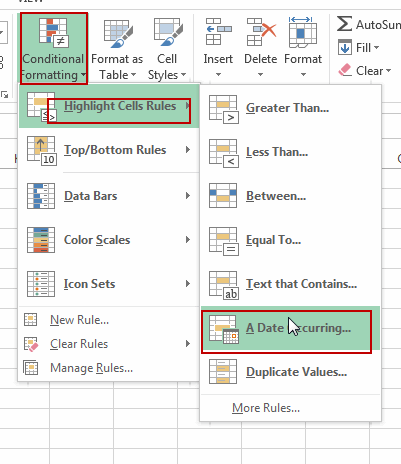#3 if you want to highlight the current day, then select Today option from the first drop down list box in the A Date Occurring dialog box, then choose one color that you want to fill the cell in the second drop down list box. Click Ok button.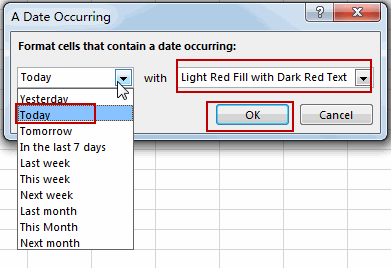Note: if you want to highlight the cell if date is in the current week, just choose This Week option from the first drop down list box. And if you want to highlight the cell if date is in the current month, just choose This Month from the first drop down list box.

#4 you should notice that the current date has been highlighted.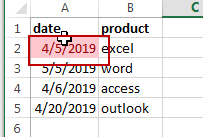## Highlight Row if Date is in Current Day/Week/Month

If you want to highlight the row that If the date in that row is equal to the current day or is in the current week or month, you can do the following steps:

#1 select the entire rows of data that contain date values.#2 go to HOME tab, click Conditional Formatting command under Styles group, Click New Rule from the drop down menu list. And the New Formatting Rule dialog will open.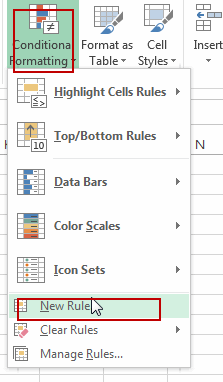#3 click Use a formula to determine which cells to format option in the New Formatting Rule dialog box, type the following formula in the Format values where this formulas is true text box.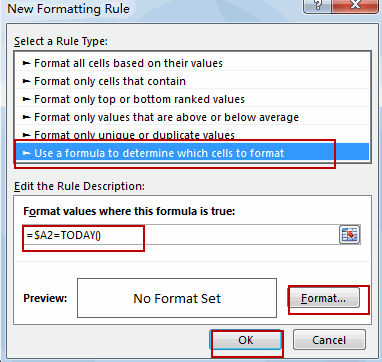To highlight current date, use the following formula:

=\$A2=TODAY()

To highlight row if date is in the current week, use the following formula:

=TODAY()-WEEKDAY(TODAY(), 3)=\$A2-WEEKDAY(\$A2, 3)

To highlight row if date is in the current month, use the following formula:

=TEXT(\$A2,”mmyy”)=TEXT(TODAY(),”mmyy”)

Note: the Cell A2 is the first cell of your selected range.

#4 click Format button, and the Format Cells dialog will open. Click Fill tab, select on color that you want to use. Click Ok button.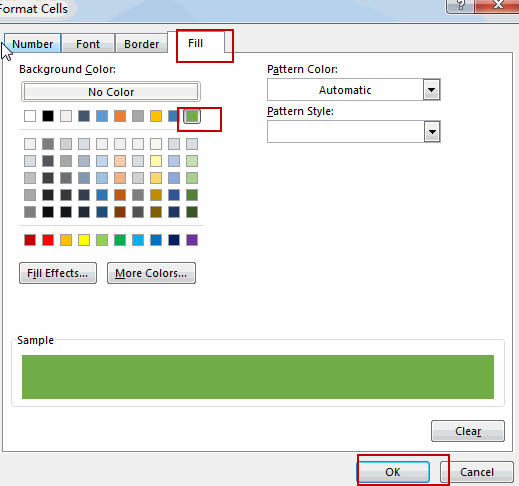#5 click Ok button. You should see that the entire rows which contain the current day have been highlighted.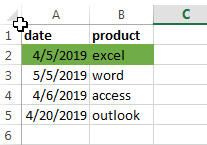### Related Functions

• Excel WEEKDAY function
The Excel WEEKDAY function returns a integer value representing the day fo the week for a given Excel date and the value is range from 1 to 7.The syntax of the WEEKDAY function is as below:=WEEKDAY (serial_number,[return_type])…
• Excel TODAY function
The Excel TODAY function returns the serial number of the current date. So you can get the current system date from the TODAY function. The syntax of the TODAY function is as below:=TODAY()…
• Excel Text function
The Excel TEXT function converts a numeric value into text string with a specified format. The TEXT function is a build-in function in Microsoft Excel and it is categorized as a Text Function. The syntax of the TEXT function is as below: = TEXT (value, Format code)…